# M/J Grade 8 Pre-Algebra (#1205070)

This document was generated on CPALMS - www.cpalms.org
You are not viewing the current course, please click the current year’s tab.

#### Course Standards

Name Description
MA.8.AR.1.1: Apply the Laws of Exponents to generate equivalent algebraic expressions, limited to integer exponents and monomial bases.
 Clarifications:Clarification 1: Refer to the K-12 Formulas (Appendix E) for the Laws of Exponents.

 Examples:The expression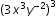is equivalent to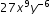.
MA.8.AR.1.2: Apply properties of operations to multiply two linear expressions with rational coefficients.
 Clarifications:Clarification 1: Problems are limited to products where at least one of the factors is a monomial. Clarification 2: Refer to Properties of Operations, Equality and Inequality (Appendix D).

 Examples:The product of (1.1+x) and (-2.3x) can be expressed as -2.53x-2.3x² or -2.3x²-2.53x.
MA.8.AR.1.3: Rewrite the sum of two algebraic expressions having a common monomial factor as a common factor multiplied by the sum of two algebraic expressions.
 Examples:The expression 99x-11x³ can be rewritten as 11x(9-x²) or as -11x(-9+x² ).
MA.8.AR.2.1: Solve multi-step linear equations in one variable, with rational number coefficients. Include equations with variables on both sides.
 Clarifications:Clarification 1: Problem types include examples of one-variable linear equations that generate one solution, infinitely many solutions or no solution.
MA.8.AR.2.2: Solve two-step linear inequalities in one variable and represent solutions algebraically and graphically.
 Clarifications:Clarification 1: Instruction includes inequalities in the forms px±q>r and p(x±q)>r, where p, q and r are specific rational numbers and where any inequality symbol can be represented. Clarification 2: Problems include inequalities where the variable may be on either side of the inequality.
MA.8.AR.2.3: Given an equation in the form of x²=p and x³=q, where p is a whole number and q is an integer, determine the real solutions.
 Clarifications:Clarification 1: Instruction focuses on understanding that when solving x²=p, there is both a positive and negative solution. Clarification 2: Within this benchmark, the expectation is to calculate square roots of perfect squares up to 225 and cube roots of perfect cubes from -125 to 125.
MA.8.AR.3.1: Determine if a linear relationship is also a proportional relationship.
 Clarifications:Clarification 1: Instruction focuses on the understanding that proportional relationships are linear relationships whose graph passes through the origin. Clarification 2: Instruction includes the representation of relationships using tables, graphs, equations and written descriptions.
MA.8.AR.3.2: Given a table, graph or written description of a linear relationship, determine the slope.
 Clarifications:Clarification 1: Problem types include cases where two points are given to determine the slope. Clarification 2: Instruction includes making connections of slope to the constant of proportionality and to similar triangles represented on the coordinate plane.
MA.8.AR.3.3: Given a table, graph or written description of a linear relationship, write an equation in slope-intercept form.
MA.8.AR.3.4: Given a mathematical or real-world context, graph a two-variable linear equation from a written description, a table or an equation in slope-intercept form.
MA.8.AR.3.5: Given a real-world context, determine and interpret the slope and y-intercept of a two-variable linear equation from a written description, a table, a graph or an equation in slope-intercept form.
 Clarifications:Clarification 1: Problems include conversions with temperature and equations of lines of fit in scatter plots.

 Examples:Raul bought a palm tree to plant at his house. He records the growth over many months and creates the equation h=0.21m+4.9, where h is the height of the palm tree in feet and m is the number of months. Interpret the slope and y-intercept from his equation.
MA.8.AR.4.1: Given a system of two linear equations and a specified set of possible solutions, determine which ordered pairs satisfy the system of linear equations.
 Clarifications:Clarification 1: Instruction focuses on the understanding that a solution to a system of equations satisfies both linear equations simultaneously.
MA.8.AR.4.2: Given a system of two linear equations represented graphically on the same coordinate plane, determine whether there is one solution, no solution or infinitely many solutions.
MA.8.AR.4.3: Given a mathematical or real-world context, solve systems of two linear equations by graphing.
 Clarifications:Clarification 1: Instruction includes approximating non-integer solutions. Clarification 2: Within this benchmark, it is the expectation to represent systems of linear equations in slope-intercept form only. Clarification 3: Instruction includes recognizing that parallel lines have the same slope.
MA.8.DP.1.1: Given a set of real-world bivariate numerical data, construct a scatter plot or a line graph as appropriate for the context.
 Clarifications:Clarification 1: Instruction includes recognizing similarities and differences between scatter plots and line graphs, and on determining which is more appropriate as a representation of the data based on the context. Clarification 2: Sets of data are limited to 20 points.

 Examples:Example: Jaylyn is collecting data about the relationship between grades in English and grades in mathematics. He represents the data using a scatter plot because he is interested if there is an association between the two variables without thinking of either one as an independent or dependent variable. Example: Samantha is collecting data on her weekly quiz grade in her social studies class. She represents the data using a line graph with time as the independent variable.
MA.8.DP.1.2: Given a scatter plot within a real-world context, describe patterns of association.
 Clarifications:Clarification 1: Descriptions include outliers; positive or negative association; linear or nonlinear association; strong or weak association.
MA.8.DP.1.3: Given a scatter plot with a linear association, informally fit a straight line.
 Clarifications:Clarification 1: Instruction focuses on the connection to linear functions. Clarification 2: Instruction includes using a variety of tools, including a ruler, to draw a line with approximately the same number of points above and below the line.
MA.8.DP.2.1: Determine the sample space for a repeated experiment.
 Clarifications:Clarification 1: Instruction includes recording sample spaces for repeated experiments using organized lists, tables or tree diagrams. Clarification 2: Experiments to be repeated are limited to tossing a fair coin, rolling a fair die, picking a card randomly from a deck with replacement, picking marbles randomly from a bag with replacement and spinning a fair spinner. Clarification 3: Repetition of experiments is limited to two times except for tossing a coin.
MA.8.DP.2.2: Find the theoretical probability of an event related to a repeated experiment.
 Clarifications:Clarification 1: Instruction includes representing probability as a fraction, percentage or decimal. Clarification 2: Experiments to be repeated are limited to tossing a fair coin, rolling a fair die, picking a card randomly from a deck with replacement, picking marbles randomly from a bag with replacement and spinning a fair spinner. Clarification 3: Repetition of experiments is limited to two times except for tossing a coin.
MA.8.DP.2.3: Solve real-world problems involving probabilities related to single or repeated experiments, including making predictions based on theoretical probability.
 Clarifications:Clarification 1: Instruction includes making connections to proportional relationships and representing probability as a fraction, percentage or decimal. Clarification 2: Experiments to be repeated are limited to tossing a fair coin, rolling a fair die, picking a card randomly from a deck with replacement, picking marbles randomly from a bag with replacement and spinning a fair spinner. Clarification 3: Repetition of experiments is limited to two times except for tossing a coin.

 Examples:Example: If Gabriella rolls a fair die 300 times, she can predict that she will roll a 3 approximately 50 times since the theoretical probability is. Example: Sandra performs an experiment where she flips a coin three times. She finds the theoretical probability of landing on exactly one head as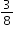. If she performs this experiment 50 times (for a total of 150 flips), predict the number of repetitions of the experiment that will result in exactly one of the three flips landing on heads.
MA.8.F.1.1: Given a set of ordered pairs, a table, a graph or mapping diagram, determine whether the relationship is a function. Identify the domain and range of the relation.
 Clarifications:Clarification 1: Instruction includes referring to the input as the independent variable and the output as the dependent variable. Clarification 2: Within this benchmark, it is the expectation to represent domain and range as a list of numbers or as an inequality.
MA.8.F.1.2: Given a function defined by a graph or an equation, determine whether the function is a linear function. Given an input-output table, determine whether it could represent a linear function.
 Clarifications:Clarification 1: Instruction includes recognizing that a table may not determine a function.
MA.8.F.1.3: Analyze a real-world written description or graphical representation of a functional relationship between two quantities and identify where the function is increasing, decreasing or constant.
 Clarifications:Clarification 1: Problem types are limited to continuous functions. Clarification 2: Analysis includes writing a description of a graphical representation or sketching a graph from a written description.
MA.8.GR.1.1: Apply the Pythagorean Theorem to solve mathematical and real-world problems involving unknown side lengths in right triangles.
 Clarifications:Clarification 1: Instruction includes exploring right triangles with natural-number side lengths to illustrate the Pythagorean Theorem. Clarification 2: Within this benchmark, the expectation is to memorize the Pythagorean Theorem. Clarification 3: Radicands are limited to whole numbers up to 225.
MA.8.GR.1.2: Apply the Pythagorean Theorem to solve mathematical and real-world problems involving the distance between two points in a coordinate plane.
 Clarifications:Clarification 1: Instruction includes making connections between distance on the coordinate plane and right triangles. Clarification 2: Within this benchmark, the expectation is to memorize the Pythagorean Theorem. It is not the expectation to use the distance formula. Clarification 3: Radicands are limited to whole numbers up to 225.

 Examples:The distance between (-2,7) and (0,6) can be found by creating a right triangle with the vertex of the right angle at the point (-2,6). This gives a height of the right triangle as 1 unit and a base of 2 units. Then using the Pythagorean Theorem the distance can be determined from the equation 1²+2²=c², which is equivalent to 5=c². So, the distance is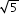units.
MA.8.GR.1.3: Use the Triangle Inequality Theorem to determine if a triangle can be formed from a given set of sides. Use the converse of the Pythagorean Theorem to determine if a right triangle can be formed from a given set of sides.
MA.8.GR.1.4: Solve mathematical problems involving the relationships between supplementary, complementary, vertical or adjacent angles.
MA.8.GR.1.5: Solve problems involving the relationships of interior and exterior angles of a triangle.
 Clarifications:Clarification 1: Problems include using the Triangle Sum Theorem and representing angle measures as algebraic expressions.
MA.8.GR.1.6: Develop and use formulas for the sums of the interior angles of regular polygons by decomposing them into triangles.
 Clarifications:Clarification 1: Problems include representing angle measures as algebraic expressions.
MA.8.GR.2.1: Given a preimage and image generated by a single transformation, identify the transformation that describes the relationship.
 Clarifications:Clarification 1: Within this benchmark, transformations are limited to reflections, translations or rotations of images. Clarification 2: Instruction focuses on the preservation of congruence so that a figure maps onto a copy of itself.
MA.8.GR.2.2: Given a preimage and image generated by a single dilation, identify the scale factor that describes the relationship.
 Clarifications:Clarification 1: Instruction includes the connection to scale drawings and proportions. Clarification 2: Instruction focuses on the preservation of similarity and the lack of preservation of congruence when a figure maps onto a scaled copy of itself, unless the scaling factor is 1.
MA.8.GR.2.3: Describe and apply the effect of a single transformation on two-dimensional figures using coordinates and the coordinate plane.
 Clarifications:Clarification 1: Within this benchmark, transformations are limited to reflections, translations, rotations or dilations of images.  Clarification 2: Lines of reflection are limited to the x-axis, y-axis or lines parallel to the axes. Clarification 3: Rotations must be about the origin and are limited to 90°, 180°, 270° or 360°. Clarification 4: Dilations must be centered at the origin.
MA.8.GR.2.4: Solve mathematical and real-world problems involving proportional relationships between similar triangles.
 Examples:During a Tampa Bay Lightning game one player, Johnson, passes the puck to his teammate, Stamkos, by bouncing the puck off the wall of the rink. The path of the puck creates two line segments that form hypotenuses for each of two similar right triangles, with the height of each triangle the distance from one of the players to the wall of the rink. If Johnson is 12 feet from the wall and Stamkos is 3 feet from the wall. How far did the puck travel from the wall of the rink to Stamkos if the distance traveled from Johnson to the wall was 16 feet?
MA.8.NSO.1.1: Extend previous understanding of rational numbers to define irrational numbers within the real number system. Locate an approximate value of a numerical expression involving irrational numbers on a number line.
 Clarifications:Clarification 1: Instruction includes the use of number line and rational number approximations, and recognizing pi (π) as an irrational number. Clarification 2: Within this benchmark, the expectation is to approximate numerical expressions involving one arithmetic operation and estimating square roots or pi (π).

 Examples:Within the expression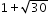, the irrational number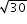can be estimated to be between 5 and 6 because 30 is between 25 and 36. By considering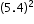and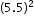, a closer approximation foris 5.5. So, the expressionis equivalent to about 6.5.
MA.8.NSO.1.2: Plot, order and compare rational and irrational numbers, represented in various forms.
 Clarifications:Clarification 1: Within this benchmark, it is not the expectation to work with the number e. Clarification 2: Within this benchmark, the expectation is to plot, order and compare square roots and cube roots. Clarification 3: Within this benchmark, the expectation is to use symbols (<, > or =).
MA.8.NSO.1.3: Extend previous understanding of the Laws of Exponents to include integer exponents. Apply the Laws of Exponents to evaluate numerical expressions and generate equivalent numerical expressions, limited to integer exponents and rational number bases, with procedural fluency.
 Clarifications:Clarification 1: Refer to the K-12 Formulas (Appendix E) for the Laws of Exponents.

 Examples:The expression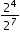is equivalent to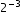which is equivalent to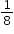.
MA.8.NSO.1.4: Express numbers in scientific notation to represent and approximate very large or very small quantities. Determine how many times larger or smaller one number is compared to a second number.
 Examples:Roderick is comparing two numbers shown in scientific notation on his calculator. The first number was displayed as 2.3147E27 and the second number was displayed as 3.5982E-5. Roderick determines that the first number is about 10³² times bigger than the second number.
MA.8.NSO.1.5: Add, subtract, multiply and divide numbers expressed in scientific notation with procedural fluency.
 Clarifications:Clarification 1: Within this benchmark, for addition and subtraction with numbers expressed in scientific notation, exponents are limited to within 2 of each other.

 Examples:The sum of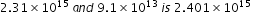.
MA.8.NSO.1.6: Solve real-world problems involving operations with numbers expressed in scientific notation.
 Clarifications:Clarification 1: Instruction includes recognizing the importance of significant digits when physical measurements are involved. Clarification 2: Within this benchmark, for addition and subtraction with numbers expressed in scientific notation, exponents are limited to within 2 of each other.
MA.8.NSO.1.7: Solve multi-step mathematical and real-world problems involving the order of operations with rational numbers including exponents and radicals.
 Clarifications:Clarification 1: Multi-step expressions are limited to 6 or fewer steps. Clarification 2: Within this benchmark, the expectation is to simplify radicals by factoring square roots of perfect squares up to 225 and cube roots of perfect cubes from -125 to 125.

 Examples:The expression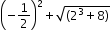is equivalent to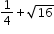which is equivalent to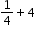which is equivalent to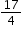.
MA.K12.MTR.1.1: Actively participate in effortful learning both individually and collectively.

Mathematicians who participate in effortful learning both individually and with others:

• Analyze the problem in a way that makes sense given the task.
• Ask questions that will help with solving the task.
• Build perseverance by modifying methods as needed while solving a challenging task.
• Stay engaged and maintain a positive mindset when working to solve tasks.
• Help and support each other when attempting a new method or approach.

 Clarifications:Teachers who encourage students to participate actively in effortful learning both individually and with others: Cultivate a community of growth mindset learners.  Foster perseverance in students by choosing tasks that are challenging. Develop students’ ability to analyze and problem solve. Recognize students’ effort when solving challenging problems.
MA.K12.MTR.2.1: Demonstrate understanding by representing problems in multiple ways.

Mathematicians who demonstrate understanding by representing problems in multiple ways:

• Build understanding through modeling and using manipulatives.
• Represent solutions to problems in multiple ways using objects, drawings, tables, graphs and equations.
• Progress from modeling problems with objects and drawings to using algorithms and equations.
• Express connections between concepts and representations.
• Choose a representation based on the given context or purpose.
 Clarifications:Teachers who encourage students to demonstrate understanding by representing problems in multiple ways: Help students make connections between concepts and representations.Provide opportunities for students to use manipulatives when investigating concepts.Guide students from concrete to pictorial to abstract representations as understanding progresses.Show students that various representations can have different purposes and can be useful in different situations.
MA.K12.MTR.3.1: Complete tasks with mathematical fluency.

Mathematicians who complete tasks with mathematical fluency:

• Select efficient and appropriate methods for solving problems within the given context.
• Maintain flexibility and accuracy while performing procedures and mental calculations.
• Complete tasks accurately and with confidence.
• Adapt procedures to apply them to a new context.
• Use feedback to improve efficiency when performing calculations.
 Clarifications:Teachers who encourage students to complete tasks with mathematical fluency:Provide students with the flexibility to solve problems by selecting a procedure that allows them to solve efficiently and accurately.Offer multiple opportunities for students to practice efficient and generalizable methods.Provide opportunities for students to reflect on the method they used and determine if a more efficient method could have been used.
MA.K12.MTR.4.1: Engage in discussions that reflect on the mathematical thinking of self and others.

Mathematicians who engage in discussions that reflect on the mathematical thinking of self and others:

• Communicate mathematical ideas, vocabulary and methods effectively.
• Analyze the mathematical thinking of others.
• Compare the efficiency of a method to those expressed by others.
• Recognize errors and suggest how to correctly solve the task.
• Justify results by explaining methods and processes.
• Construct possible arguments based on evidence.
 Clarifications:Teachers who encourage students to engage in discussions that reflect on the mathematical thinking of self and others:Establish a culture in which students ask questions of the teacher and their peers, and error is an opportunity for learning.Create opportunities for students to discuss their thinking with peers.Select, sequence and present student work to advance and deepen understanding of correct and increasingly efficient methods.Develop students’ ability to justify methods and compare their responses to the responses of their peers.
MA.K12.MTR.5.1: Use patterns and structure to help understand and connect mathematical concepts.

Mathematicians who use patterns and structure to help understand and connect mathematical concepts:

• Focus on relevant details within a problem.
• Create plans and procedures to logically order events, steps or ideas to solve problems.
• Decompose a complex problem into manageable parts.
• Relate previously learned concepts to new concepts.
• Look for similarities among problems.
• Connect solutions of problems to more complicated large-scale situations.
 Clarifications:Teachers who encourage students to use patterns and structure to help understand and connect mathematical concepts:Help students recognize the patterns in the world around them and connect these patterns to mathematical concepts.Support students to develop generalizations based on the similarities found among problems.Provide opportunities for students to create plans and procedures to solve problems.Develop students’ ability to construct relationships between their current understanding and more sophisticated ways of thinking.
MA.K12.MTR.6.1: Assess the reasonableness of solutions.

Mathematicians who assess the reasonableness of solutions:

• Estimate to discover possible solutions.
• Use benchmark quantities to determine if a solution makes sense.
• Check calculations when solving problems.
• Verify possible solutions by explaining the methods used.
• Evaluate results based on the given context.
 Clarifications:Teachers who encourage students to assess the reasonableness of solutions:Have students estimate or predict solutions prior to solving.Prompt students to continually ask, “Does this solution make sense? How do you know?”Reinforce that students check their work as they progress within and after a task.Strengthen students’ ability to verify solutions through justifications.
MA.K12.MTR.7.1: Apply mathematics to real-world contexts.

Mathematicians who apply mathematics to real-world contexts:

• Connect mathematical concepts to everyday experiences.
• Use models and methods to understand, represent and solve problems.
• Perform investigations to gather data or determine if a method is appropriate. • Redesign models and methods to improve accuracy or efficiency.
 Clarifications:Teachers who encourage students to apply mathematics to real-world contexts:Provide opportunities for students to create models, both concrete and abstract, and perform investigations.Challenge students to question the accuracy of their models and methods.Support students as they validate conclusions by comparing them to the given situation.Indicate how various concepts can be applied to other disciplines.
ELA.K12.EE.1.1: Cite evidence to explain and justify reasoning.
 Clarifications:K-1 Students include textual evidence in their oral communication with guidance and support from adults. The evidence can consist of details from the text without naming the text. During 1st grade, students learn how to incorporate the evidence in their writing.2-3 Students include relevant textual evidence in their written and oral communication. Students should name the text when they refer to it. In 3rd grade, students should use a combination of direct and indirect citations.4-5 Students continue with previous skills and reference comments made by speakers and peers. Students cite texts that they’ve directly quoted, paraphrased, or used for information. When writing, students will use the form of citation dictated by the instructor or the style guide referenced by the instructor. 6-8 Students continue with previous skills and use a style guide to create a proper citation.9-12 Students continue with previous skills and should be aware of existing style guides and the ways in which they differ.
ELA.K12.EE.2.1: Read and comprehend grade-level complex texts proficiently.
 Clarifications:See Text Complexity for grade-level complexity bands and a text complexity rubric.
ELA.K12.EE.3.1: Make inferences to support comprehension.
 Clarifications:Students will make inferences before the words infer or inference are introduced. Kindergarten students will answer questions like “Why is the girl smiling?” or make predictions about what will happen based on the title page. Students will use the terms and apply them in 2nd grade and beyond.
ELA.K12.EE.4.1: Use appropriate collaborative techniques and active listening skills when engaging in discussions in a variety of situations.
 Clarifications:In kindergarten, students learn to listen to one another respectfully.In grades 1-2, students build upon these skills by justifying what they are thinking. For example: “I think ________ because _______.” The collaborative conversations are becoming academic conversations.In grades 3-12, students engage in academic conversations discussing claims and justifying their reasoning, refining and applying skills. Students build on ideas, propel the conversation, and support claims and counterclaims with evidence.
ELA.K12.EE.5.1: Use the accepted rules governing a specific format to create quality work.
 Clarifications:Students will incorporate skills learned into work products to produce quality work. For students to incorporate these skills appropriately, they must receive instruction. A 3rd grade student creating a poster board display must have instruction in how to effectively present information to do quality work.
ELA.K12.EE.6.1: Use appropriate voice and tone when speaking or writing.
 Clarifications:In kindergarten and 1st grade, students learn the difference between formal and informal language. For example, the way we talk to our friends differs from the way we speak to adults. In 2nd grade and beyond, students practice appropriate social and academic language to discuss texts.
ELD.K12.ELL.MA.1: English language learners communicate information, ideas and concepts necessary for academic success in the content area of Mathematics.
ELD.K12.ELL.SI.1: English language learners communicate for social and instructional purposes within the school setting.

## General Course Information and Notes

### VERSION DESCRIPTION

The benchmarks in this course are mastery goals that students are expected to attain by the end of the year. To build mastery, students will continue to review and apply earlier grade-level benchmarks and expectations.

### GENERAL NOTES

In grade 8, instructional time will emphasize six areas: (1) representing numbers in scientific notation and extending the set of numbers to the system of real numbers, which includes irrational numbers; (2) generate equivalent numeric and algebraic expressions including using the Laws of Exponents; (3) creating and reasoning about linear relationships including modeling an association in bivariate data with a linear equation; (4) solving linear equations, inequalities and systems of linear equations; (5) developing an understanding of the concept of a function and (6) analyzing two-dimensional figures, particularly triangles, using distance, angle and applying the Pythagorean Theorem.

Curricular content for all subjects must integrate critical-thinking, problem-solving, and workforce-literacy skills; communication, reading, and writing skills; mathematics skills; collaboration skills; contextual and applied-learning skills; technology-literacy skills; information and media-literacy skills; and civic-engagement skills.

English Language Development ELD Standards Special Notes Section:
Teachers are required to provide listening, speaking, reading and writing instruction that allows English language learners (ELL) to communicate information, ideas and concepts for academic success in the content area of Mathematics. For the given level of English language proficiency and with visual, graphic, or interactive support, students will interact with grade level words, expressions, sentences and discourse to process or produce language necessary for academic success. The ELD standard should specify a relevant content area concept or topic of study chosen by curriculum developers and teachers which maximizes an ELL's need for communication and social skills. To access an ELL supporting document which delineates performance definitions and descriptors, please click on the following link:

### General Information

 Course Number: 1205070 Course Path: Section: Grades PreK to 12 Education Courses > Grade Group: Grades 6 to 8 Education Courses > Subject: Mathematics > SubSubject: General Mathematics > Abbreviated Title: M/J GRADE 8 PRE-ALG Course Attributes: Florida Standards Course Course Type: Core Academic Course Course Level: 2 Course Status: State Board Approved

#### State Adopted Instructional Materials

 Carnegie Learning FL Middle School Math Solution, Grade 8 Mathematics: Pre-AlgebraSandy Bartle Finocchi and Amy Jones Lewis - Carnegie Learning, Inc. dba EMC Publishing & Mondo Ed - 1st - 2023enVision Florida B.E.S.T. Mathematics Grade 8 Pre-AlgebraBerry, et al - Savvas Learning Company LLC, formerly known as Pearson K12 Learning LLC. - 1 - 2023Florida EdGems Math Course 3Shannon McCaw - EdGems Math LLC - 1st - 2022Florida Reveal Math, Grade 8 Pre-AlgebraCathy L. Seeley , Ed.D; Raj Shah, Ph.D.; Cheryl R. Tobey, M.Ed.; Dinah Zike, M.Ed.; Walter Secada, Ph.D. - McGraw Hill LLC - 1 - 2023Florida's B.E.S.T. Standards for MATH Grade 8 Pre-Algebra with CalcChat® and CalcView®Ron Larson and Laurie Boswell - Big Ideas Learning, LLC - 1 - 2023HMH Florida's B.E.S.T. Into MathEdward B. Burger, PhD; Juli K. Dixon, PhD; Timothy D. Kanold, PhD; Matthew R. Larson, PhD; Steven J. Leinwand, PhD - Houghton Mifflin Harcourt - N/A - 2023Math Nation: Florida's B.E.S.T. 8th Grade Math: Pre-AlgebraMath Nation - Math Nation (a division of Study Edge) - 1 - 2023